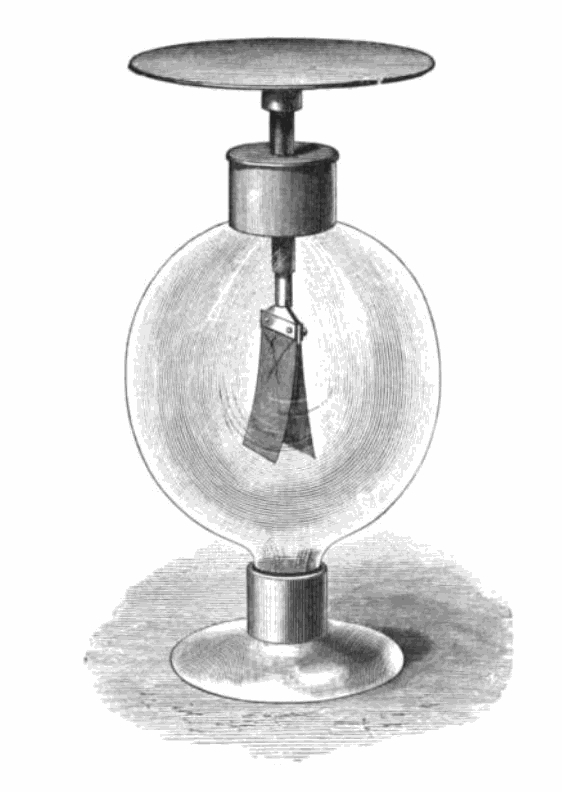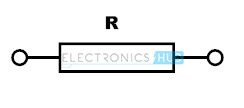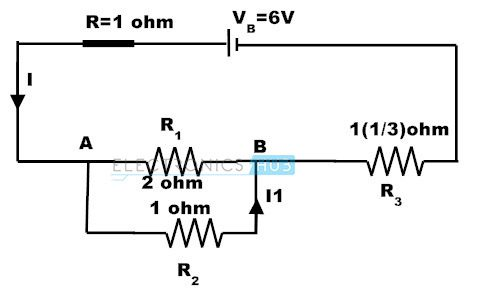## Home# Electricity Basics

Outline

### Static Electricity- Its Perspective and Development

Mr. Theophrastus in 321BC observed that the power of amber attracts straws, dry leaves after it has been rubbed with a woolen cloth. This was the first observation of electricity and was the first recorded experiment.

Later in 1600 BC, Dr.Gilbert discovered that this attraction could be excited in many other substances besides amber. He is considered as the founder of the Electrical science.

Mr.Otto Guericke invented the first electrical machine in the year 1674. He made it by utilizing a ball of sulphur fixing it on a spindle to turn it with a handle. A friction was created by pressing the ball with a finger or hand. This machine first produced the electric spark with sound. This turned the science to make wonders to originate a new science. In this experiment, it was also observed that the ball which was excited and charged for a short time attraction will be repelled by the same object.

Sir Isaac Newton in 1675 constructed the same experiment done by Mr.Otto Guericke with some changes. Sir Newton used a glass globe and this globe produced the sparks of ½ inch and could feel them with the finger placing on it.

Later Mr. Stephen Grey in 1720 further developed that the electricity may be conveyed from the machine to the body by a wire or wet thread at a considerable distance.

In the year 1733, Mr.M.Dufay further experimented and found that if the body is kept on a stand made up of glass rods could be excited if the glass rods kept dry. In this experiment, it was also conferred that there are two kinds of electricity one which was excited by that of glass and the other excited by resin, silks etc.

Later in 1736, the Newton’s experiment improved by Professor Winckler. He utilized the horse hair covered with silk cloth instead of using the fingers or hand to rub the glass ball. In 1738 Professor Boze discovered that keeping a tube of metal can collect this electricity. It was also found that the electricity will disappear if there are sharp edges or points on this metal conductor.

Dr.Grey made several trails with different kinds of matter and discovered that some bodies will have the power to conduct electricity and some of them will not.

With all the experiments, the discovery of an electrical machine has been done with the observations, improvements and innovative developments done by these scientists with individual or combined efforts. But the amount of power produced is in small quantity. There is an effort of several scientific investigators who are responsible for inventing the great Leyden’s jar. Over a period of time with many improvements and developments this led to a great product called ‘battery’.

BACK TO TOP

### Static Electricity

Static electricity is the subject which deals with the study of electric charges at rest.

Recalling the above experiments done by or tried by the ancient eminent scientists, a spark or sparks have been produced with the help of glass, felt or with some other material. In all of these cases the movement of electron is responsible for this phenomenon. As per this elementary electro-static behavior, the entire process is the movement of electrons from one body to the other, thereby electrically charging of these bodies. One of the bodies will be charged positively (deficiency of electrons) and the other one negatively (excess of electrons).

BACK TO TOP

#### Properties of the Electrical Bodies

Recalling the experiment of Mr. Dufay, two glass rods fixing on wooden stand as shown in the figure with a crossbar on them.From the center of the crossbar a wire strip should be suspended by a silk thread, so that the wire strip will be able to move freely in a horizontal plane like a compass needle. A silk handkerchief is also needed to excite the glass rods. The experiment has to be in a warm dry room.

Now, after rubbing the glass rods briskly with the handkerchief, (it is better if two people with two handkerchiefs do the experiment) placing one at the stirred up and holding the other at the rubbed end of the wire strip. It will be observed that if the two glass rods are taken, a strong repulsion will take place and if one glass rod taken, an attraction will take place.

So, it can be concluded that similar electrifications will repel each other and unlike electrifications attracts. For this reason there exist two kinds of electricity:  (+) and (-) electricity, the electricity of the glass will be +ve electricity. By adding equal quantities of the glass electricity is zero. This resembles an algebraic sum of + and – which is equal to zero. The same experiment can be carried out by replacing the wire strip with a feather. The feather will be attracted to the rods.

Like the above experiment if the one end of the glass rod is electrified it remains for a considerable time keeping the other end un-electrified. In the same way, if one end of a metal rod is electrified, it will be distributed along the length of the rod and if the other end is connected to ground, it vanishes.

BACK TO TOP

### Gold Leaf Electroscope

This is the oldest instrument used to detect and determines the electrification.`Image Resource Link: upload.wikimedia.org/wikipedia/commons/b/b3/Gold_leaf_electroscope_1869.png`

As shown in the figure, two gold leaves will be hanged (fixed one side) and attached to a metal rod which was fixed to a brass plate through a hole placed in a glass shade. When an electric body was placed on this plate, a portion of the electricity will pass through the gold leaves charging them to repel each other.  This is the measure of the charge. This is a very sensitive instrument used to detect small quantities of the electricity.

BACK TO TOP

### Electro-static Induction

When a charged body is placed near another body without any physical contact between them, the second body will get electrified. The charge will exist on the other body till the charged body was present near to it, but as soon as the charged body is withdrawn the charge on the second body will disappear. This is called as electrification by induction. For example, if we rub the both hands together and place it on the above gold leaf electroscope, the gold leaves will be repelled, but as soon we remove our hands from the plate of the Gold leaf electroscope, the leaves will come to normal position.

BACK TO TOP

### Faraday’s Ice Pail Experiment

A charged sphere was placed into a hollow cylinder which was on the plate of the electroscope. The gold leaves spread apart till the sphere is in touch position; the leaves retained the original position as soon as the ball is removed.

This shows that

• Equal and opposite charges will be produced by induction.
• The charge cannot reside inside the hollow cylinder.

BACK TO TOP

### Laws of Electrical Forces

The force of attraction or repulsion between two electrified bodies varies inversely to the square of their distance apart where the body sizes are very small compared with their distance.

BACK TO TOP

### Electric Field and Potential:

An electric field is a region of space in which electric charges activated by electric forces.

The basic unit of electric charge is the coulomb.

Like gravitation and magnetic fields the electric field strength is measured in terms of force on a unit positive charge.

Electric field strength is defined as the force acting on one coulomb of positive charge at that point. The direction of the field will be in the direction of the positive charge movement.

Breakdown field strength is the field strength which causes the insulator to break down. The breakdown field strength of air is 3 x106 volts/meter which limits the maximum potential of the conductor. Beyond this point corona discharge will occur.

BACK TO TOP

### Electrical Potential Difference

This is analogous to natural potential energy. A mass lifted against gravitational force in the gravitational field will be given potential energy. In the same way a charge can be given electrical potential energy by being moved against the force excited on it in an electric field.

The volt is defined as the potential difference between two points such that one joule of work is done if one coulomb of positive charge is moved from one point to the other.

The change in electrical energy is W of a charge q when moved with a potential difference of one volt is given by

W = qV

The electric potential at a point is defined as the work done for moving or bringing a unit of positive charge from infinity to the point.

BACK TO TOP

### Electric Circuits

An electric circuit is a closed path which allows the electrons to flow through it continuously. If the circuit is broken, then the current flow cannot occur through it. The location of a break in the circuit is not a matter. A break, anywhere in the circuit prevents the flow of current through the circuit.

BACK TO TOP

### Voltage and Current

The electric current is the rate of flow of charge. The coulomb is the practical unit measurement of electric charge in MKS systems. One unit of charge carries 6.28 x1018 electrons. So, the rate of charge transfer of one coulomb per second is called ‘ampere’.

Current I = Q/t

Where Q is charge and measured in coulombs,

t is time and it measured in seconds.

If the electron flow is not steady or moving in a non-uniform motion this ampere can be written as

Instantaneous current I = rate of change of average charge (coulombs) = dq/dt

In the same way we can say the net charge of current Q = ∫ i dt which is the integration of current.

Example-1:

A charge of 4000 coulombs passes a point P in electric circuit for half an hour. What is the average current flow?

I = Q/t = 4000/1800 = 2.2 coulombs/sec; t = 30 mins = 30×60 = 1800 sec

Example-2:

The observed current flow in the circuit is 100mA. How much charge is transferred in 5 minutes?

Q = I x t = (100/1000) x (5 x 60) = 30 coulombs

Example-3:

Typical values of copper are

n = 8.5 x 1028 m-3

e = 1.6 x 10-19C

I = 10 amps

A = 10-6 m2

the velocity of charges

v = I/A.n.e = 10 / (10-6 x 8.5 x 1028 x 1.6 x 10-19) = 7.3 x 10-3 m/s.

BACK TO TOP

#### Electric Voltage:

It must be noted that an energy source is required to introduce the energy into the system. This energy source acts on the charge carriers, giving rise to current. Some of these sources are battery cells, generators, fuel cells and solar cells. That means a source will have the power to generate the electro-motive-force (emf). The emf is defined as the source of energy which can deliver the power to pass the charge through the source to form an electrical circuit. The unit of emf is volt.

Vab= W / Q

where

Vab is the potential difference in volts between points a and b

Q = amount of charge in coulombs moving from a to b

W = energy level difference of the charge as it moves from a to b.

The energy will be measured in Joules. So, 1 joule of energy is required to move 1 coulomb of charge for getting 1 volt of voltage.

BACK TO TOP

### Resistance

In the electric circuit whatever may be the source of the energy it was connected, the electrons will flow through the circuit elements where the electric current will take place. The circuit elements are mainly made of solids and the electrons will flow through them. The solids will contain different structures of atoms and while the electrons travelling through these elements; they will collide with the atoms and molecules. These collisions affect the flow of electrons because of the resistance offered by the atoms. This resistance differs from material to the material and the flow of electrons depends on the property of the material which was in the circuit. Hence, the resistance is the property of that particular material which opposes the flow of electrons. The device which is having the property of electrons opposition as the main property is called as “Resistor”. The symbol of the resistor is given below.Unit of Resistance is ohm and it is designated with a letter ’Ω’

A resistance of one ohm in a particular wire or material is that if it develops 0.24 calories of heat when one ampere of current flows through it for 1 second.

#### Ohm’s Law:

In the year 1827, George Simon Ohm, a scientist observed that the electrical direct current (D.C.) flowing through a metallic conductor varies directly with the voltage applied to it. This is called the ohm’s law. According to the law

Current, I = [Voltage (V)/Resistance (R)] in Amperes (A).

Therefore

Resistance R = (V/I) ohms

BACK TO TOP

### Conductance:

The conductance of the material is the converse of the resistive property of that material. It means the conductance is the reciprocal of the resistance in the mathematical form. The symbol of conductance is G and is equal to 1/R.

The unit of conductance is siemen and will be denoted as ‘℧’.

If 1 volt of the voltage is applied to a conductor and if 1 ampere current flows through it for a second, the material or wire is having a conductance of 1 mho.

BACK TO TOP

### Voltage and Current in a Practical Circuit

Any practical electrical current source will have an internal resistance. Depending upon the origin of source generation this internal resistance varies. For example in a generator the internal resistance is the resistance of the copper windings. In practice it cannot be removed. The potential difference between the two terminals of the voltage source when the source (E) is idle never matches with that of the potential difference when the source is delivering the electric current (V). This is the result of the internal resistance and difference of these potential differences is called as lost volts (E – V). Obviously this is the voltage drop of the internal resistance (E –V = Ir)Example:

If the internal resistance of the battery is 1 ohm, Calculate

a) Voltage across the resistor R3,

b) current through R1,

c) lost volts as per the following circuit.Sol:

The resultant resistance of R1, Rwill be Ro

Ro = (R1 x R2) / (R1 + R2) = 2/3 ohms

The total resistance = Ro +R3 + battery internal resistance = 2/3 + 1(1/3) + 1 = 3 ohms.

Current from the battery = 6/3 = 2 amps

a) Voltage across the resistor R3 = 2 x 1(1/3) = 2(2/3) V

b) Voltage across Ro = 2 x 2/3 = 4/3 = 1(1/3) V,

Current through R1 = V/R1 = (4/3)/2 =2/3 A

c) Lost volts= I x r = 2 x 1 = 2 V

Finally by cross checking: E = Vr + VR1 + VR3 = 2 + 1(1/3) +2(2/3) = 6 V.

BACK TO TOP

NEXT – ELECTRICAL SAFETY TIPS

### One Response

1.gary fry says:

Thanks for posting. Thought it would be beneficial for you to correct these contradicting statements:

Mr. Theophrastus in 321BC observed that the power of amber attracts straws, dry leaves after it has been rubbed with a woolen cloth. This was the first observation of electricity and was the first recorded experiment.

Later in 1600 BC, Dr.Gilbert discovered that this attraction could be excited in many other substances besides amber. He is considered as the founder of the Electrical science.

How did Dr. Gilbert know about Mr T’s observations 900ish years earlier?